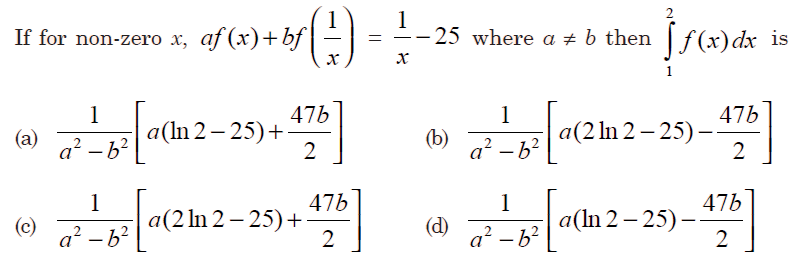# GATE | GATE-CS-2015 (Set 3) | Question 55(A) A
(B) B
(C) C
(D) D

Explanation: Given-Puttingwe get-Subtracting the second equation from the first one we get-Putting this value back in the first equation-Now that we havewe can findTherefore the correct option is A.

Quiz of this Question